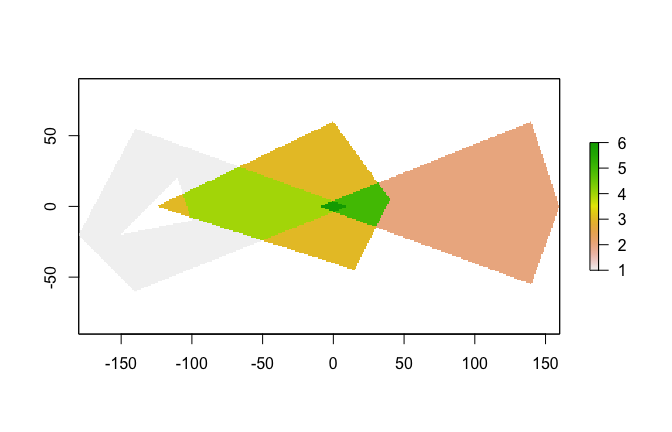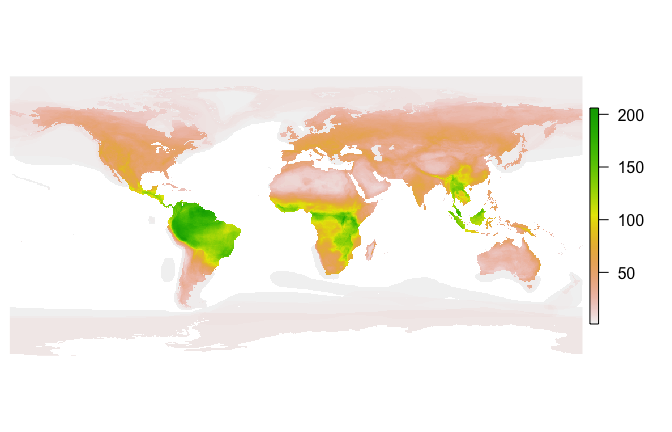# Using Fasterize

#### 2018-03-21

fasterize is high-performance replacement for the rasterize() function in the raster package.

Functionality is currently limited to rasterizing polygons in sf-type data frames.

## Installation

Install the current version of fasterize from CRAN:

install.packages('fasterize')

Install the development version of fasterize with devtools:

devtools::install_github("ecohealthalliance/fasterize")

fasterize uses Rcpp and thus requires a compile toolchain to install from source. Testing (and most use) requires sf, which requires GDAL (>= 2.0.0), GEOS (>= 3.3.0), and PROJ.4 (>= 4.8.0) to be installed on your system.

## Usage

The main function, fasterize(), takes the same inputs as raster::rasterize() but currently has fewer options and is is limited to rasterizing polygons.

A method for creating empty rasters from sf objects is provided, and raster plot methods are re-exported.

library(raster)
library(fasterize)
library(sf)
p1 <- rbind(c(-180,-20), c(-140,55), c(10, 0), c(-140,-60), c(-180,-20))
hole <- rbind(c(-150,-20), c(-100,-10), c(-110,20), c(-150,-20))
p1 <- list(p1, hole)
p2 <- list(rbind(c(-10,0), c(140,60), c(160,0), c(140,-55), c(-10,0)))
p3 <- list(rbind(c(-125,0), c(0,60), c(40,5), c(15,-45), c(-125,0)))
pols <- st_sf(value = c(1,2,3),
geometry = st_sfc(lapply(list(p1, p2, p3), st_polygon)))
r <- raster(pols, res = 1)
r <- fasterize(pols, r, field = "value", fun="sum")
plot(r)## Performance

Let’s compare fasterize() to raster::rasterize():

pols_r <- as(pols, "Spatial")
bench <- microbenchmark::microbenchmark(
rasterize = r <- raster::rasterize(pols_r, r, field = "value", fun="sum"),
fasterize = f <- fasterize(pols, r, field = "value", fun="sum"),
unit = "ms"
)
print(bench, digits = 3)
#> Unit: milliseconds
#>       expr     min     lq    mean  median     uq    max neval cld
#>  rasterize 340.376 359.69 379.197 369.552 386.65 497.83   100   b
#>  fasterize   0.337   0.37   0.545   0.414   0.64   2.51   100  a

How does fasterize() do on a large set of polygons? Here I download the IUCN shapefile for the ranges of all terrestrial mammals and generate a 1/6 degree world map of mammalian biodiversity by rasterizing all the layers.

if(!dir.exists("Mammals_Terrestrial")) {
"https://s3.amazonaws.com/hp3-shapefiles/Mammals_Terrestrial.zip",
destfile = "Mammals_Terrestrial.zip") # <-- 383 MB
unzip("Mammals_Terrestrial.zip", exdir = ".")
}
mammal_shapes <- st_read("Mammals_Terrestrial")
#> Reading layer Mammals_Terrestrial' from data source /Users/noamross/dropbox-eha/projects-eha/fasterize/Mammals_Terrestrial' using driver ESRI Shapefile'
#> Simple feature collection with 42714 features and 27 fields
#> geometry type:  MULTIPOLYGON
#> dimension:      XY
#> bbox:           xmin: -180 ymin: -85.58276 xmax: 180 ymax: 89.99999
#> epsg (SRID):    4326
#> proj4string:    +proj=longlat +datum=WGS84 +no_defs
mammal_raster <- raster(mammal_shapes, res = 1/6)
bench2 <- microbenchmark::microbenchmark(
mammals = mammal_raster <- fasterize(mammal_shapes, mammal_raster, fun="sum"),
times=20, unit = "s")
print(bench2, digits=3)
#> Unit: seconds
#>     expr   min    lq  mean median    uq   max neval
#>  mammals 0.856 0.883 0.903  0.904 0.922 0.954    20
par(mar=c(0,0.5,0,0.5))
plot(mammal_raster, axes=FALSE, box=FALSE)`